CBSE (Science) Class 11CBSE
Share

# HC Verma solutions for Class 11 Physics chapter 20 - Photoelectric Effect and Wave-Particle Duality

#### HC Verma Concepts of Physics - Vol. 2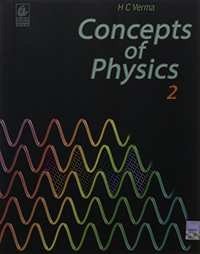## Chapter 20: Photoelectric Effect and Wave-Particle Duality

#### HC Verma solutions for Class 11 Physics Chapter 20 Exercise Short Answers, short Answers [Page 363]

Short Answers | Q 1 | Page 363

Can we find the mass of a photon by the definition p = mv?

Short Answers | Q 2 | Page 363

Is it always true that for two sources of equal intensity, the number of photons emitted in a given time are equal?

Short Answers | Q 3 | Page 363

What is the speed of a photon with respect to another photon if (a) the two photons are going in the same direction and (b) they are going in opposite directions?

Short Answers | Q 4 | Page 363

Can a photon be deflected by an electric field? Or by a magnetic field?

Short Answers | Q 5 | Page 363

A hot body is placed in a closed room maintained at a lower temperature. Is the number of photons in the room increasing?

short Answers | Q 6 | Page 363

Should the energy of a photon be called its kinetic energy or its internal energy?

Short Answers | Q 7 | Page 363

In an experiment on photoelectric effect, a photon is incident on an electron from one direction and the photoelectron is emitted almost in the opposite direction. Does this violate the principle of conservation of momentum?

Short Answers | Q 8 | Page 363

It is found that yellow light does not eject photoelectrons from a metal. Is it advisable to try with orange light or with green light?

Short Answers | Q 9 | Page 363

It is found that photosynthesis starts in certain plants when exposed to sunlight, but it does not start if the plants are exposed only to infrared light. Explain.

Short Answers | Q 10 | Page 363

The threshold wavelength of a metal is λ0. Light of wavelength slightly less than λ0 is incident on an insulated plate made of this metal. It is found that photoelectrons are emitted for some time and after that the emission stops. Explain.

Short Answers | Q 11 | Page 363

Is p − E/c valid for electrons?

Short Answers | Q 12 | Page 363

Consider the de-Broglie wavelength of an electron and a proton. Which wavelength is smaller if the two particles have (a) the same speed (b) the same momentum (c) the same energy?

Short Answers | Q 13 | Page 363

If an electron has a wavelength, does it also have a colour?

#### HC Verma solutions for Class 11 Physics Chapter 20 Exercise MCQ [Pages 264 - 364]

MCQ | Q 1 | Page 363

Planck's constant has the same dimensions as

• force × time

• force × distance

• force × speed

• force × distance time

MCQ | Q 2 | Page 363

Two photons of

• equal wavelength have equal linear momenta

• equal energies have equal linear momenta

• equal frequencies have equal linear momenta

• equal linear momenta have equal wavelengths

MCQ | Q 3 | Page 363

Let p and E denote the linear momentum and energy of a photon. If the wavelength is decreased,

•  both p and E increase

• p increases and E decreases

• p decreases and E increases

•  both p and E decrease

MCQ | Q 4 | Page 363

Let nr and nb be the number of photons emitted by a red bulb and a blue bulb, respectively, of equal power in a given time.

• nr = nb

• nr < nb

• nr > nb

• The information is insufficient to derive a relation between nr and nb.

MCQ | Q 5 | Page 363

The equation E = pc is valid

• for an electron as well as for a photon

• for an electron but not for a photon

• for a photon but not for an electron

• neither for an electron nor for a photon

MCQ | Q 6 | Page 363

The work function of a metal is hv0. Light of frequency v falls on this metal. Photoelectric effect will take place only if

• v ≥ v0

• v > 2v0

• v < v0

• v < v0/2

MCQ | Q 7 | Page 363

Light of wavelength λ falls on a metal with work-function hc/λ0. Photoelectric effect will take place only if

• λ ≥ λ0

•  λ ≥ 2λ0

• λ ≤ λ0

• λ < λ0/2

MCQ | Q 8 | Page 364

When stopping potential is applied in an experiment on photoelectric effect, no photoelectric is observed. This means that

• the emission of photoelectrons is stopped

• the photoelectrons are emitted but are re-absorbed

• the photoelectrons are accumulated near the collector plate

• the photoelectrons are dispersed from the sides of the apparatus

MCQ | Q 9 | Page 364

If the frequency of light in a photoelectric experiment is doubled, the stopping potential will

• be doubled

• be halved

• become more than double

• become less than double

MCQ | Q 10 | Page 364

The frequency and intensity of a light source are doubled. Consider the following statements.

(A) The saturation photocurrent remains almost the same.
(B) The maximum kinetic energy of the photoelectrons is doubled.

• A and B are true.

• A is true but B is false.

• A is false but B is true.

• A and B are false.

MCQ | Q 11 | Page 364

A point source of light is used in a photoelectric effect. If the source is removed farther from the emitting metal, the stopping potential

• will increase

• will decrease

• will remain constant

• will either increase or decrease

MCQ | Q 12 | Page 364

A point source causes photoelectric effect from a small metal plate. Which of the following curves may represent the saturation photocurrent as a function of the distance between the source and the metal?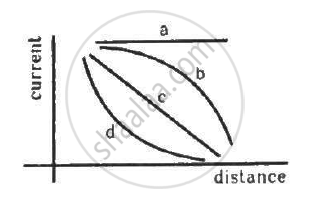• a

• b

• c

• d

MCQ | Q 13 | Page 264

A non-monochromatic light is used in an experiment on photoelectric effect. The stopping potential

• is related to the mean wavelength

•  is related to the longest wavelength

• is related to the shortest wavelength

• is not related to the wavelength

MCQ | Q 14 | Page 264

A proton and an electron are accelerated by the same potential difference. Let λe and λpdenote the de Broglie wavelengths of the electron and the proton, respectively.

• λe = λp

• λe < λp

• λe > λp

• The relation between λe and λp depends on the accelerating potential difference.

#### HC Verma solutions for Class 11 Physics Chapter 20 Exercise MCQ [Pages 264 - 364]

MCQ | Q 1 | Page 264

When the intensity of a light source in increased,
(a) the number of photons emitted by the source in unit time increases
(b) the total energy of the photons emitted per unit time increases
(c) more energetic photons are emitted
(d) faster photons are emitted

MCQ | Q 2 | Page 364

Photoelectric effect supports quantum nature of light because
(a) there is a minimum frequency below which no photoelectrons are emitted
(b) the maximum kinetic energy of photoelectrons depends only on the frequency of light and not on its intensity
(c) even when the metal surface is faintly illuminated the photoelectrons leave the surface immediately
(d) electric charge of the photoelectrons is quantised

MCQ | Q 3 | Page 364

A photon of energy hv is absorbed by a free electron of a metal with work-function hv − φ.

• The electron is sure to come out.

• The electron is sure to come out with kinetic energy hv − φ.

• Either the electron does not come out or it comes our with kinetic energy hv − φ.

• It may come out with kinetic energy less than hv − φ.

MCQ | Q 4 | Page 364

If the wavelength of light in an experiment on photoelectric effect is doubled,
(a) photoelectric emission will not take place
(b) photoelectric emission may or may not take place
(c) the stopping potential will increase
(d) the stopping potential will decrease

MCQ | Q 5 | Page 364

The photo current in an experiment on photoelectric effect increases if

• the intensity of the source is increased

• the exposure time is increased

• the intensity of the source is decreased

• the exposure time is decreased

MCQ | Q 6 | Page 364

The collector plate in an experiment on photoelectric effect is kept vertically above the emitter plate. A light source is put on and a saturation photocurrent is recorded. An electric field is switched on that has a vertically downward direction.

• The photocurrent will increase.

• The kinetic energy of the electrons will increase.

• The stopping potential will decrease.

• The threshold wavelength will increase.

MCQ | Q 7 | Page 364

In which of the following situations, the heavier of the two particles has smaller de Broglie wavelength? The two particles
(a) move with the same speed
(b) move with the same linear momentum
(c) move with the same kinetic energy
(d) have fallen through the same height

#### HC Verma solutions for Class 11 Physics Chapter 20 [Pages 365 - 366]

Q 1 | Page 365

Visible light has wavelengths in the range of 400 nm to 780 nm. Calculate the range of energy of the photons of visible light.

(Use h = 6.63 × 10-34J-s = 4.14 × 10-15 eV-s, c = 3 × 108 m/s and me = 9.1 × 10-31kg)

Q 2 | Page 365

Calculate the momentum of a photon of light of wavelength 500 nm.

(Use h = 6.63 × 10-34J-s = 4.14 × 10-15 eV-s, c = 3 × 108 m/s and me = 9.1 × 10-31kg)

Q 3 | Page 365

An atom absorbs a photon of wavelength 500 nm and emits another photon of wavelength 700 nm. Find the net energy absorbed by the atom in the process.

(Use h = 6.63 × 10-34J-s = 4.14 × 10-15 eV-s, c = 3 × 108 m/s and me = 9.1 × 10-31kg)

Q 4 | Page 365

Calculate the number of photons emitted per second by a 10 W sodium vapour lamp. Assume that 60% of the consumed energy is converted into light. Wavelength of sodium light = 590 nm

(Use h = 6.63 × 10-34J-s = 4.14 × 10-15 eV-s, c = 3 × 108 m/s and me = 9.1 × 10-31kg)

Q 5 | Page 365

When the sun is directly overhead, the surface of the earth receives 1.4 × 103 W m−2 of sunlight. Assume that the light is monochromatic with average wavelength 500 nm and that no light is absorbed in between the sun and the earth's surface. The distance between the sun and the earth is 1.5 × 1011 m. (a) Calculate the number of photons falling per second on each square metre of earth's surface directly below the sun. (b) How many photons are there in each cubic metre near the earth's surface at any instant? (c) How many photons does the sun emit per second?

(Use h = 6.63 × 10-34J-s = 4.14 × 10-15 eV-s, c = 3 × 108 m/s and me = 9.1 × 10-31kg)

Q 6 | Page 365

A parallel beam of monochromatic light of wavelength 663 nm is incident on a totally reflecting plane mirror. The angle of incidence is 60° and the number of photons striking the mirror per second is 1.0 × 1019. Calculate the force exerted by the light beam on the mirror.

(Use h = 6.63 × 10-34J-s = 4.14 × 10-15 eV-s, c = 3 × 108 m/s and me = 9.1 × 10-31kg)

Q 7 | Page 365

A beam of white light is incident normally on a plane surface absorbing 70% of the light and reflecting the rest. If the incident beam carries 10 W of power, find the force exerted by it on the surface.

(Use h = 6.63 × 10-34J-s = 4.14 × 10-15 eV-s, c = 3 × 108 m/s and me = 9.1 × 10-31kg)

Q 8 | Page 365

A totally reflecting, small plane mirror placed horizontally faces a parallel beam of light, as shown in the figure. The mass of the mirror is 20 g. Assume that there is no absorption in the lens and that 30% of the light emitted by the source goes through the lens. Find the power of the source needed to support the weight of the mirror.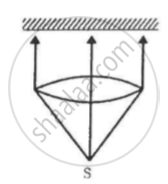(Use h = 6.63 × 10-34J-s = 4.14 × 10-15 eV-s, c = 3 × 108 m/s and me = 9.1 × 10-31kg)

Q 9 | Page 365

A 100 W light bulb is placed at the centre of a spherical chamber of radius 20 cm. Assume that 60% of the energy supplied to the bulb is converted into light and that the surface of the chamber is perfectly absorbing. Find the pressure exerted by the light on the surface of the chamber.

(Use h = 6.63 × 10-34J-s = 4.14 × 10-15 eV-s, c = 3 × 108 m/s and me = 9.1 × 10-31kg)

Q 10 | Page 365

A sphere of radius 1.00 cm is placed in the path of a parallel beam of light of large aperture. The intensity of the light is 0.5 W cm−2. If the sphere completely absorbs the radiation falling on it, find the force exerted by the light beam on the sphere.

(Use h = 6.63 × 10-34J-s = 4.14 × 10-15 eV-s, c = 3 × 108 m/s and me = 9.1 × 10-31kg)

Q 11 | Page 365

A sphere of radius 1.00 cm is placed in the path of a parallel beam of light of large aperture. The intensity of the light is 0.5 W cm−2. If the sphere completely absorbs the radiation falling on it, Show that the force on the sphere due to the light falling on it is the same even if the sphere is not perfectly absorbing.

Q 12 | Page 365

Show that it is not possible for a photon to be completely absorbed by a free electron.

Q 13 | Page 365

Two neutral particles are kept 1 m apart. Suppose by some mechanism some charge is transferred from one particle to the other and the electric potential energy lost is completely converted into a photon. Calculate the longest and the next smaller wavelength of the photon possible.

(Use h = 6.63 × 10-34J-s = 4.14 × 10-15 eV-s, c = 3 × 108 m/s and me = 9.1 × 10-31kg)

Q 14 | Page 365

Find the maximum kinetic energy of the photoelectrons ejected when light of wavelength 350 nm is incident on a cesium surface. Work function of cesium = 1.9 eV

(Use h = 6.63 × 10-34J-s = 4.14 × 10-15 eV-s, c = 3 × 108 m/s and me = 9.1 × 10-31kg)

Q 15 | Page 365

The work function of a metal is 2.5 × 10−19 J. (a) Find the threshold frequency for photoelectric emission. (b) If the metal is exposed to a light beam of frequency 6.0 × 1014 Hz, what will be the stopping potential?

(Use h = 6.63 × 10-34J-s = 4.14 × 10-15 eV-s, c = 3 × 108 m/s and me = 9.1 × 10-31kg)

Q 16 | Page 365

The work function of a photoelectric material is 4.0 eV. (a) What is the threshold wavelength? (b) Find the wavelength of light for which the stopping potential is 2.5 V.

(Use h = 6.63 × 10-34J-s = 4.14 × 10-15 eV-s, c = 3 × 108 m/s and me = 9.1 × 10-31kg)

Q 17 | Page 365

Find the maximum magnitude of the linear momentum of a photoelectron emitted when a wavelength of 400 nm falls on a metal with work function 2.5 eV.

(Use h = 6.63 × 10-34J-s = 4.14 × 10-15 eV-s, c = 3 × 108 m/s and me = 9.1 × 10-31kg)

Q 18 | Page 365

When a metal plate is exposed to a monochromatic beam of light of wavelength 400 nm, a negative potential of 1.1 V is needed to stop the photo current. Find the threshold wavelength for the metal.

(Use h = 6.63 × 10-34J-s = 4.14 × 10-15 eV-s, c = 3 × 108 m/s and me = 9.1 × 10-31kg)

Q 19 | Page 365

In an experiment on photoelectric effect, the stopping potential is measured for monochromatic light beams corresponding to different wavelengths. The data collected are as follows:-

Wavelength (nm):         350   400   450   500   550
Stopping potential (V): 1.45  1.00  0.66  0.38  0.16

Plot the stopping potential against inverse of wavelength (1/λ) on a graph paper and find (a) Planck's constant (b) the work function of the emitter and (c) the threshold wavelength.

(Use h = 6.63 × 10-34J-s = 4.14 × 10-15 eV-s, c = 3 × 108 m/s and me = 9.1 × 10-31kg)

Q 20 | Page 365

The electric field associated with a monochromatic beam is 1.2 × 1015 times per second. Find the maximum kinetic energy of the photoelectrons when this light falls on a metal surface whose work function is 2.0 eV.

(Use h = 6.63 × 10-34J-s = 4.14 × 10-15 eV-s, c = 3 × 108 m/s and me = 9.1 × 10-31kg)

Q 21 | Page 365

The electric field associated with a light wave is given by E = E_0 sin [(1.57 xx 10^7  "m"^-1)(x - ct)]. Find the stopping potential when this light is used in an experiment on photoelectric effect with the emitter having work function 1.9 eV.

(Use h = 6.63 × 10-34J-s = 4.14 × 10-15 eV-s, c = 3 × 108 m/s and me = 9.1 × 10-31kg)

Q 22 | Page 366

The electric field at a point associated with a light wave is E = (100  "Vm"^-1) sin [(3.0 xx 10^15 "s"^-1)t] sin [(6.0 xx 10^15 "s"^-1)t].If this light falls on a metal surface with a work function of 2.0 eV, what will be the maximum kinetic energy of the photoelectrons?

(Use h = 6.63 × 10-34J-s = 4.14 × 10-15 eV-s, c = 3 × 108 m/s and me = 9.1 × 10-31kg)

Q 23 | Page 366

A monochromatic light source of intensity 5 mW emits 8 × 1015 photons per second. This light ejects photoelectrons from a metal surface. The stopping potential for this setup is 2.0 V. Calculate the work function of the metal.

(Use h = 6.63 × 10-34J-s = 4.14 × 10-15 eV-s, c = 3 × 108 m/s and me = 9.1 × 10-31kg)

Q 24 | Page 366

The figure is the plot of stopping potential versus the frequency of the light used in an experiment on photoelectric effect. Find (a) the ratio h/e and (b) the work function.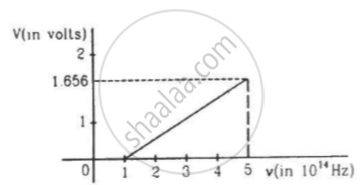Q 25 | Page 366

A photographic film is coated with a silver bromide layer. When light falls on this film, silver bromide molecules dissociate and the film records the light there. A minimum of 0.6 eV is needed to dissociate a silver bromide molecule. Find the maximum wavelength of light that can be recorded by the film.

(Use h = 6.63 × 10-34J-s = 4.14 × 10-15 eV-s, c = 3 × 108 m/s and me = 9.1 × 10-31kg)

Q 26 | Page 366

In an experiment on photoelectric effect, light of wavelength 400 nm is incident on a cesium plate at the rate of 5.0 W. The potential of the collector plate is made sufficiently positive with respect to the emitter, so that the current reaches its saturation value. Assuming that on average, one out of every 106 photons is able to eject a photoelectron, find the photocurrent in the circuit.

Q 27 | Page 366

A silver ball of radius 4.8 cm is suspended by a thread in a vacuum chamber. Ultraviolet light of wavelength 200 nm is incident on the ball for some time during which light energy of 1.0 × 10−7 J falls on the surface. Assuming that on average, one photon out of every ten thousand is able to eject a photoelectron, find the electric potential at the surface of the ball, assuming zero potential at infinity. What is the potential at the centre of the ball?

(Use h = 6.63 × 10-34J-s = 4.14 × 10-15 eV-s, c = 3 × 108 m/s and me = 9.1 × 10-31kg)

Q 28 | Page 366

In an experiment on photoelectric effect, the emitter and the collector plates are placed at a separation of 10 cm and are connected through an ammeter without any cell. A magnetic field B exists parallel to the plates. The work function of the emitter is 2.39 eV and the light incident on it has wavelengths between 400 nm and 600 nm. Find the minimum value of B for which the current registered by the ammeter is zero. Neglect any effect of space charge.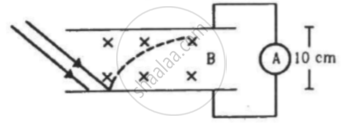Q 29 | Page 366

In the arrangement shown in the figure, y = 1.0 mm, d = 0.24 mm and D = 1.2 m. The work function of the material of the emitter is 2.2 eV. Find the stopping potential V needed to stop the photocurrent.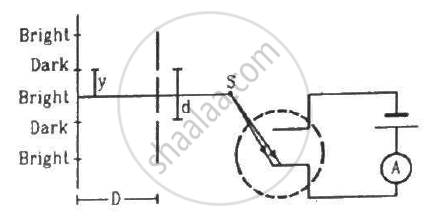(Use h = 6.63 × 10-34J-s = 4.14 × 10-15 eV-s, c = 3 × 108 m/s and me = 9.1 × 10-31kg)

Q 30 | Page 366

In a photoelectric experiment, the collector plate is at 2.0 V with respect to the emitter plate made of copper (φ = 4.5 eV). The emitter is illuminated by a source of monochromatic light of wavelength 200 nm. Find the minimum and maximum kinetic energy of the photoelectrons reaching the collector.

Q 31 | Page 366

A small piece of cesium metal (φ = 1.9 eV) is kept at a distance of 20 cm from a large metal plate with a charge density of 1.0 × 10−9 C m−2 on the surface facing the cesium piece. A monochromatic light of wavelength 400 nm is incident on the cesium piece. Find the minimum and maximum kinetic energy of the photoelectrons reaching the large metal plate. Neglect any change in electric field due to the small piece of cesium present.

(Use h = 6.63 × 10-34J-s = 4.14 × 10-15 eV-s, c = 3 × 108 m/s and me = 9.1 × 10-31kg)

Q 32 | Page 366

Consider the situation of the previous problem. Consider the faster electron emitted parallel to the large metal plate. Find the displacement of this electron parallel to its initial velocity before it strikes the large metal plate.

(Use h = 6.63 × 10-34J-s = 4.14 × 10-15 eV-s, c = 3 × 108 m/s and me = 9.1 × 10-31kg)

Q 33 | Page 366

A horizontal cesium plate (φ = 1.9 eV) is moved vertically downward at a constant speed v in a room full of radiation of wavelength 250 nm and above. What should be the minimum value of v so that the vertically-upward component of velocity is non-positive for each photoelectron?

(Use h = 6.63 × 10-34J-s = 4.14 × 10-15 eV-s, c = 3 × 108 m/s and me = 9.1 × 10-31kg)

Q 34 | Page 366

A small metal plate (work function φ) is kept at a distance d from a singly-ionised, fixed ion. A monochromatic light beam is incident on the metal plate and photoelectrons are emitted. Find the maximum wavelength of the light beam, so that some of the photoelectrons may go round the ion along a circle.

Q 35 | Page 366

A light beam of wavelength 400 nm is incident on a metal plate of work function 2.2 eV. (a) A particular electron absorbs a photon and makes two collisions before coming out of the metal. Assuming that 10% of the extra energy is lost to the metal in each collision, find the kinetic energy of this electron as it comes out of the metal. (b) Under the same assumptions, find the maximum number of collisions the electron can suffer before it becomes unable to come out of the metal.

## Chapter 20: Photoelectric Effect and Wave-Particle Duality

#### HC Verma Concepts of Physics - Vol. 2## HC Verma solutions for Class 11 Physics chapter 20 - Photoelectric Effect and Wave-Particle Duality

HC Verma solutions for Class 11 Physics chapter 20 (Photoelectric Effect and Wave-Particle Duality) include all questions with solution and detail explanation. This will clear students doubts about any question and improve application skills while preparing for board exams. The detailed, step-by-step solutions will help you understand the concepts better and clear your confusions, if any. Shaalaa.com has the CBSE Concepts of Physics - Vol. 2 solutions in a manner that help students grasp basic concepts better and faster.

Further, we at Shaalaa.com provide such solutions so that students can prepare for written exams. HC Verma textbook solutions can be a core help for self-study and acts as a perfect self-help guidance for students.

Concepts covered in Class 11 Physics chapter 20 Photoelectric Effect and Wave-Particle Duality are Einstein’S Photoelectric Equation: Energy Quantum of Radiation, Particle Nature of Light: the Photon, Photoelectric Effect and Wave Theory of Light, Experimental Study of Photoelectric Effect, Einstein’s Equation - Particle Nature of Light, Electron Emission, Davisson-Germer Experiment, de-Broglie Relation, Wave Nature of Matter, Photoelectric Effect - Hallwachs’ and Lenard’S Observations, Photoelectric Effect - Hertz’S Observations, Dual Nature of Radiation.

Using HC Verma Class 11 solutions Photoelectric Effect and Wave-Particle Duality exercise by students are an easy way to prepare for the exams, as they involve solutions arranged chapter-wise also page wise. The questions involved in HC Verma Solutions are important questions that can be asked in the final exam. Maximum students of CBSE Class 11 prefer HC Verma Textbook Solutions to score more in exam.

Get the free view of chapter 20 Photoelectric Effect and Wave-Particle Duality Class 11 extra questions for Physics and can use Shaalaa.com to keep it handy for your exam preparation

S